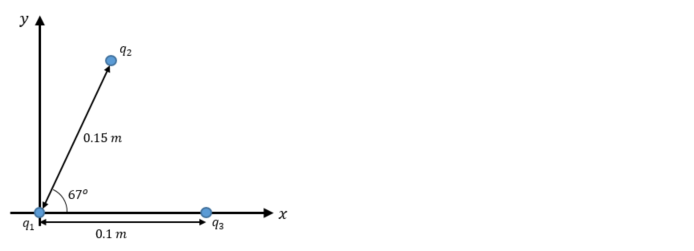# Problem: Find the magnitude and direction of the net electrostatic force on q 1 due to q 2 and q3 as shown in the figure. The charges are q 1 = +4.0 μC, q2 = -6.0 μC, q3 = -5.0 μC.

###### FREE Expert Solution
87% (295 ratings)
###### Problem Details

Find the magnitude and direction of the net electrostatic force on q 1 due to q 2 and q3 as shown in the figure. The charges are q 1 = +4.0 μC, q2 = -6.0 μC, q3 = -5.0 μC.